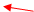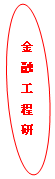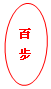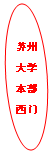## 2019年金融数学与金融工程青年学者研讨会

1.参会代表免交注册费、提供午餐和晚餐，承担参会代表的住宿费。

2.如需要，会议支付参会代表的往返差旅费

 姓名 单位 郭洁 苏州科技大学 吕思宇 东南大学 黄伟桓 山东大学 林建伟 莆田学院 马成虎 复旦大学 马俊美 上海财经大学 穆蕊 苏州大学 钱晓松 苏州大学 钱林义 华东师范大学 秦聪 苏州大学 石芸 华东师范大学 宋颖达 上海交通大学 王过京 苏州大学 万相伟 上海交通大学 徐玉红 苏州大学 杨淑振 山东大学 岳兴业 苏州大学 郑国强 东南大学 卓小杨 清华大学五道口金融学院

 时间 主持人 报告人 报告题目 9月25日 报到注册 9月26日 8:30-9:00 签到、领取会议材料 9:00-9:30 徐玉红 马成虎 不完备市场下的福利经济学定理及汇总问题 9:35-10:05 钱林义 Valuation   of Risk-Based Premium of DB Pension Plan with Terminations 10:05-10:35 合影 茶歇 10:35-11:05 钱晓松 林建伟 Optimal   dividend strategy with endogenous bankruptcy boundary under Chapter 11 of the   US bankruptcy code 11:10-11:40 吕思宇 Optimal   Switching under a Hybrid Diffusion Model and Applications to Stock Trading 12:00-14:00 午餐 14:00-14:30 穆蕊 石芸 When is Systematic   Risk Priced? A New Perspective from Probability Weighting 14:35-15:05 马俊美 Lévy   模型下金融衍生品定价的重要抽样技术研究 15:05-15:35 茶歇 15:35-16:05 吕思宇 郑国强 Absence of   dominating probability for weakly compact probability variety 16:10-16:40 郭洁 Pricing   default risk in mortgage-backed securities under a regime-switching   reduced-form model 17:30 晚宴 9月27日 9:00-9:30 杨淑振 万相伟 Hermite Expansion   for Transition Densities of Irreducible Diffusions with An Application to   Option Pricing 9:35-10:05 宋颖达 Optimal Stopping   with Random Lag 10:05-10:35 茶歇 10:35-11:05 郑国强 杨淑振 Stochastic optimal   control problem with varying terminal time and related application 11:10-11:40 卓小杨 The R Measures: A   Theory of Expected Returns of Contingent Claims 12:00-14:00 午餐

Valuation of Risk-Based Premium of DB Pension Plan with Terminations

Optimal dividend strategy with endogenous bankruptcy boundary under Chapter 11 of the US bankruptcy code

This paper concerns the optimal dividend strategy problem with endogenous bankruptcy boundary under the presence of Chapter 11 of the US bankruptcy code. For any given declaring bankruptcy boundary under Chapter 11, we model the value function as the solution to a singular stochastic control problem and its corresponding closed-form solution is obtained by partial differential equation. Meanwhile, we prove that the optimal dividend strategy for any given bankruptcy boundary is a barrier strategy constructed by a unique reflecting barrier. The optimal bankruptcy boundary is numerically determined by maximizing the value function. Numerical results show that Chapter 11 has a role impact on the optimal dividend strategy problem. The company can get the bigger total value by filing for Chapter 11; The company pays dividends to shareholders earlier to maximize the total value of the company, but the expected liquidation time is shorter.

Optimal Switching under a Hybrid Diffusion Model and Applications to Stock Trading

This talk is concerned with the optimal switching problem under a hybrid diffusion (or, regime switching) model in an infinite horizon. The state of the system consists of a number of diffusions coupled by a finite-state continuous-time Markov chain. Based on the dynamic programming principle, the value function of our optimal switching problem is proved to be the unique viscosity solution to the associated system of variational inequalities. The optimal switching strategy, indicating when and where it is optimal to switch, is given in terms of the switching and continuation regions. In many applications, the underlying Markov chain has a large state space and exhibits two-time-scale structure. In this case, a singular perturbation approach is employed to reduce the computational complexity involved. It is shown that as the time-scale parameter $\varepsilon$ goes to zero, the value function of the original problem converges to that of a limit problem. The limit problem is much easier to solve, and its optimal switching solution leads to an approximate solution to the original problem. Finally, as an application of our theoretical results, an example concerning the stock trading problem in a regime switching market is provided. It is emphasized that, this paper is the first time to introduce the optimal switching as a general framework to study the stock trading problem, in view of their inherent connection. Both optimal trading rule and convergence result are numerically demonstrated in this example. This is a joint work with Profs Zhen Wu and Qing Zhang.

When is Systematic Risk Priced? A New Perspective from Probability Weighting

The central task of asset pricing is to examine how expected returns are related to risk. Probability weighting affects investor's perception of risk, especially the tail risk. This paper wants to examine the role of probability weighting behind asset pricing, both theoretically and empirically.On the theoretical front, we consider a one-period-two-date economy with rank-dependent utility agents. After deriving the equilibrium asset pricing formula under probability weighting, we show that in the derived three-moment-CAPM model, the existence of probability weighting is helpful in understanding both beta anomaly and coskewness pricing. On the empirical front, we first develop several indices to identify the probability weighting levels and further separate the market into two regimes: overweighting periods and underweighting periods. We find that stock's expected return is positively related to its beta risk during overweighting periods, but negatively related to its beta risk during underweighting periods. Unconditional on probability weighting, these two effects offset each other, resulting in a flat relationship between beta and return. We also find that the stock's expected return is negatively related to its co-skewness during overweighting periods but unrelated to its co-skewness during underweighting periods.

Lévy 模型下金融衍生品定价的重要抽样技术研究

Absence of dominating probability for weakly compact probability variety

Based on the Halmos-Savage theorem, a new tractable criterion isconfirmed on recognizing whether a family of probabilities can be dominated by a single probability or not, the implementation of which to the probability variety involved in $G$-expectation evinces the absence of a dominating probability. Therefore even though the static model of $G$-expectation can be constructed with the help of countably infinitely many probabilities those can be dominated simultaneously, when it comes to dynamic situation, such dominating probability measure does not exist. The key ingredient of our proof relies on the normability of Wasserstein metric of order one inprobability space.

Pricing default risk in mortgage-backed securities under a regime-switching reduced-form model

In this paper, we propose a reduced-form model to price default risk in mortgages and fixed-rate pass-through mortgage-backed securities. Since the default of borrowers is often affected by macroeconomic conditions, we take into account the changes of market regimes. We introduce a default factor process to model the default risk of borrowers and define its exponential decreasing rate as default rate, which follows a shot-noise process. The model assumes that the interest rate and the default rate are all influenced by macroeconomic conditions described by a homogeneous Markov chain. Explicit pricing formulas for mortgage-backed securities with defaultable underlying mortgages are obtained through the conditional Laplace transform of the regime-switching shot-noise process. Numerical illustration is also presented to show the impact of model parameters on the price.

Hermite Expansion for Transition Densities of Irreducible Diffusions with An Application to Option Pricing

A diffusion is said to be reducible if there exists a one-to-one transformation of the diffusion into a new one whose diffusion matrix is the identity matrix, otherwise it is irreducible. Most multivariate diffusions such as the stochastic volatility models are irreducible. As pointed out by Ait-Sahalia (2008), the straight Hermite expansion of Ait-Sahalia (2002) will not in general converge for irreducible diffusions.

In this paper we manage to develop the Hermite expansion for transition densities of irreducible diffusions, which converges as the time interval shrinks to zero. By introducing a quasi-Lamperti transform unitizing the process' diffusion matrix at the initial time, we can expand the transition density of the transformed process using Hermite polynomials as the orthogonal basis. Then we derive explicit recursive formulas for the expansion coefficients using the It\^{o}-Taylor expansion method, and prove the small-time convergence of the expansion. Moreover, we show that the derived Hermite expansion unifies some existing methods including the expansions of Li (2013) and Yang, Chen and Wan (2019). In addition, we demonstrate the advantage of Hermite expansion by deriving explicit recursive expansion formulas for European option prices under irreducible diffusions. Numerical experiments illustrate the accuracy and effectiveness of our approach.

Optimal Stopping with Random Lag

This paper is concerned with the optimal stopping problem with random time lag under general Markov processes. We solve the problem by constructing a continues-time Markov chain (CTMC) to approximate the general Markov process model and compute the solution under the CTMC. We prove the convergence our method. Based on our method, we analyze the impact of time lag with various distributions on the optimal stopping problem.

Stochastic optimal control problem with varying terminal time and related application

To establish the stochastic maximum principle for the optimal control problem under state constraints without using Ekland's variational principle, we propose a new optimal control problem, in which the terminal time follows the varying of the control via the constrained condition. Focusing on this new optimal control problem, we investigate a novel stochastic maximum principle, which differ from the traditional optimal control problem under state constraints.The optimal pair of the optimal control model can be verified via this new stochastic maximum principle. In addition, this new optimal control model can not only minimize the cost functional but also decreases the holding time.

The R Measures: A Theory of Expected Returns of Contingent Claims

This paper introduces a new probability measure R for deriving the analytical solution of a contingent claim's expected price at a given finite horizon H, less than or equal to the terminal date T, on which the claim pays out a single risky cash flow. Using the R measure, we show that any model that admits an analytical solution to a contingent claim's current price will generally provide an analytical solution to the claim's expected price at the horizon H. The R measure is a hybrid measure that nests both the physical probability measure P and the risk-neutral measure Q. We also extend the R measure to RT forward measure for deriving analytical solutions of a contingent claim's expected price at a given finite horizon, when the short rate is stochastic. We present some stylized facts about the term structure of expected returns and the horizon structure of expected returns of contingent claims such as equity options, Treasuary bonds, bond options, and corporate bonds. This is a joint work with Sanjay K. Nawalkha (University of Massachusetts).

（外地赴苏州可选择乘高铁至苏州高铁北站或苏州站，或乘飞机至上海虹桥机场

 南林饭店 苏州高铁北站 地铁苏州高铁北站乘地铁2号线（桑田岛方向）至苏州火车站换乘，换乘地铁4号线（同里方向）至三元坊站下车。从1号口出站，步行727米到达。 打车约52元 苏州站 地铁苏州站乘地铁4号线（同里方向）至三元坊站。从1号口出站，步行727米到达。 打车约18元 上海虹桥机场 高铁从上海虹桥火车站乘坐高铁至苏州高铁北站或苏州站（约27分钟）。然后按上述两种方式至南林饭店。 打车约300元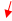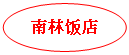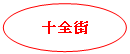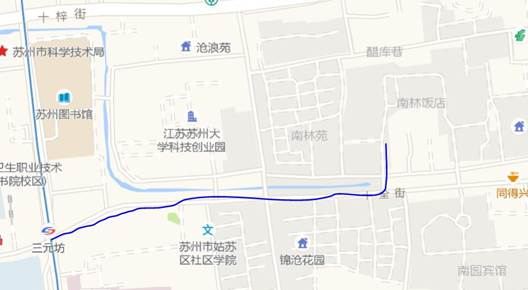15分钟1.3公里）